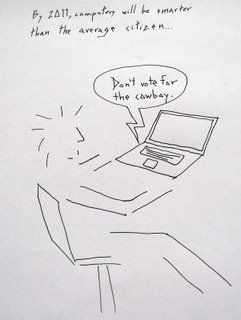### Computability: theoretical or practical?

Alan Turing defined in 1936 the now well known "Turing Machine" to define computation. A Turing Machine is actually an abstract mathematical construction, which cannot be fully materialized, since it requires an infinite tape (memory). Nevertheless, people have been discussing their brains out on whether the human mind (whatever that may be) is computable or not. This is because people believe that if whatever our brains or minds do is computable, then (in principle) it could be modelled in a computer, thus reaching the ultimate goal of artificial intelligence: a machine with the same intellectual abilities as a human (whatever that may be).

Still, computability as we understand it, i.e. Turing computability, i.e. a function that a Turing machine can perform, is only computability in theory. In practice, there are Turing-computable numbers that are not computable in practice by our computers, since there would not be enough time in the universe to calculate them, or memory to store them. And there are also non-Turing computable operations (such as the halting function of a Turing Machine) that are computable in practice (for the case of halting functions, cheating with an "oracle", i.e. knowledge about the machine outside the machine).

So, to conclude, I believe we should really distinguish Turing computation (calculated by abstract Turing machines) from pragmatic computation (calculated by any actual computing device). Then we can subdivide pragmatic computation depending on the device itself, i.e. there will be functions pragmatically computable with an abacus, some more with a PC, some others with DNA, some others with a brain...

P.S. For those interested on whether a digital computer (or robot) will be able to perform the same functions as a human, the answer is: we'll know if it's possible only when we see it... (which is actually what the Turing Test (1950) proposed...)